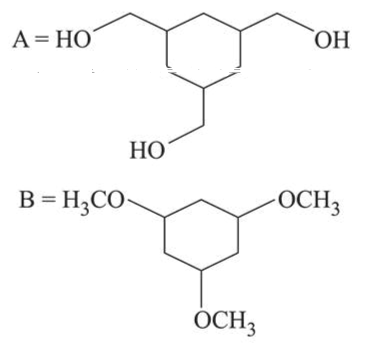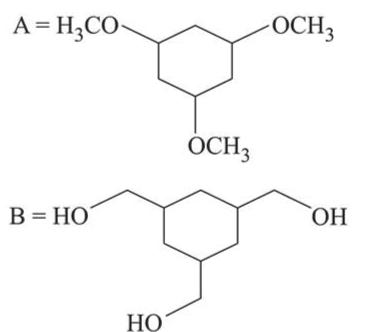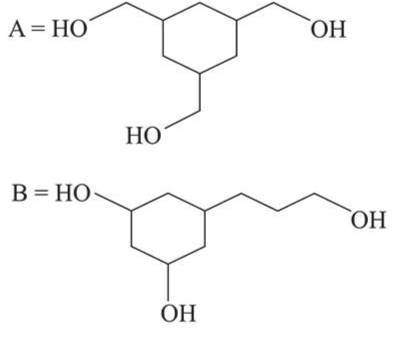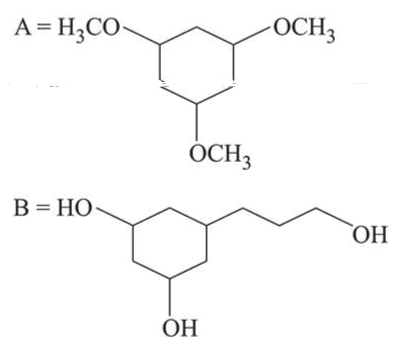# Among the compounds A and B with molecular formula

Question:

Among the compounds $A$ and $B$ with molecular formula $\mathrm{C}_{9} \mathrm{H}_{18} \mathrm{O}_{3}, A$ is having higher boiling point the $B$. The possible structures of $A$ and $B$ are:

1.2.3.4.Correct Option: 1

Solution:

In (A), $-\mathrm{OH}$ group is present, so inter-molecular H-bonding is possible while in (B), due to methoxy group there is no possibility of Inter-molecular H-bonding. So $\mathrm{A}$ is having higher boiling point than B.